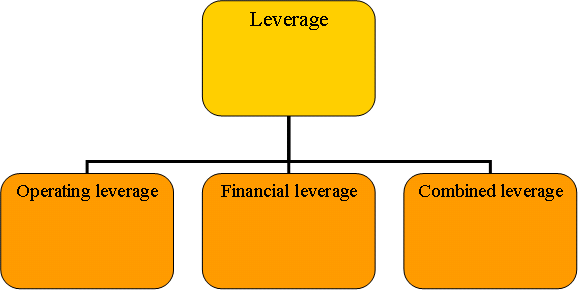# Leverage analysis and types

A lever is a force in a car or in any machine which helps in doing more work with lesser labor. In financial management leverage analysis means arranging fixed assets in such a way that fixed return is ensured. The types of leverage analysis are:

1.) Operating leverage: firm generally purchases the assets, that its operation will produce revenue. When sale increases the fixed cost remains the same and operating revenue will increases. As fixed cost is constant, the % change in operating revenue is more than % change in sale. Hence there is a positive relation between operating leverage and break even point.

Operating leverage= % change in operating profit/ % change in sales

2.) Financial leverage: it is also called trading on equity. Financial leverage means the use of preference share capital, equity share capital along with fixed interest bearing securities or debentures.

Financial leverage= % change in earning per share/ % change in earning before interest and tax

Financial leverage assumes that the firm is capable of earning more on assets than that acquired by use of funds, on which fixed rate of dividend is paid.3.) Combined leverage: it measures the total leverage due to the both operating and financial leverage.

Combined leverage= % change in earning per share/ % change in sales

Thus, to conclude we can say that in operating leverage change in sale have effect on EBIT; in financial leverage change in EBIT have effect on EPS.

So the firm uses proper amount of both operating leverage and financial leverage as even a small change in sale changes EPS.

EPS increase if sale increases and EPS decreases if sale decreases; there is a positive relation between the two.

I hope you understood leverage analysis.